Ex 1.2

Chapter 1 Class 12 Relation and Functions
Serial order wise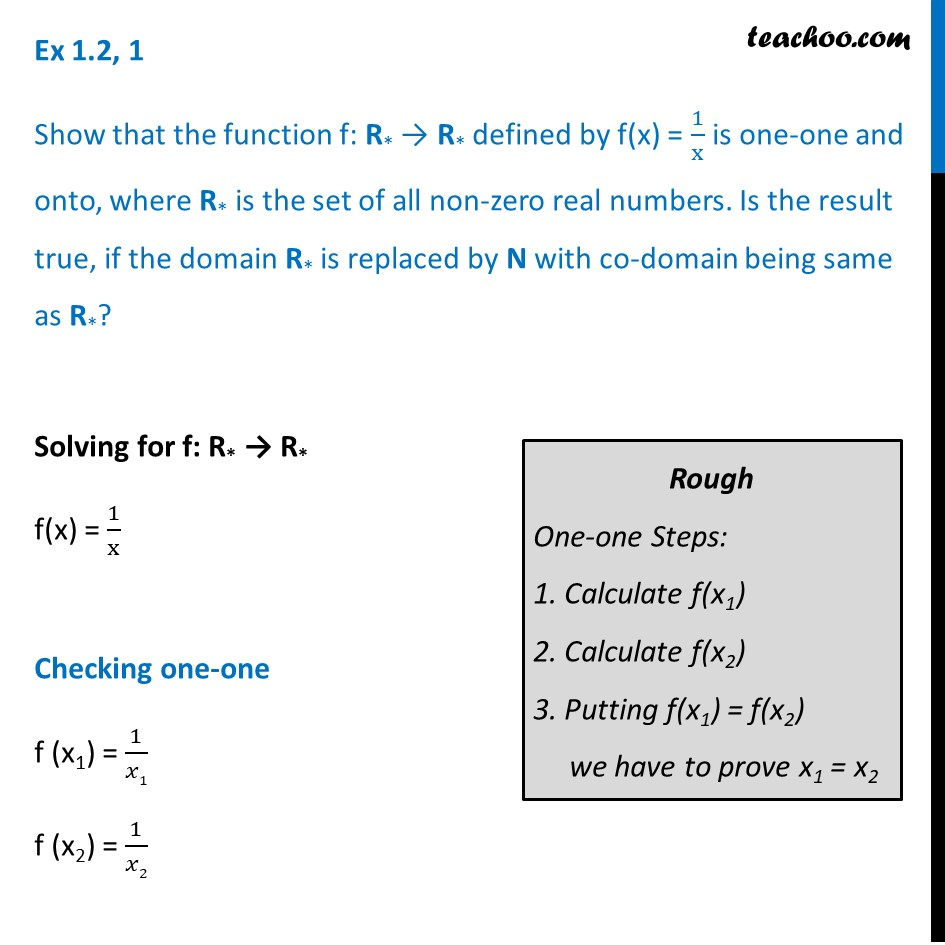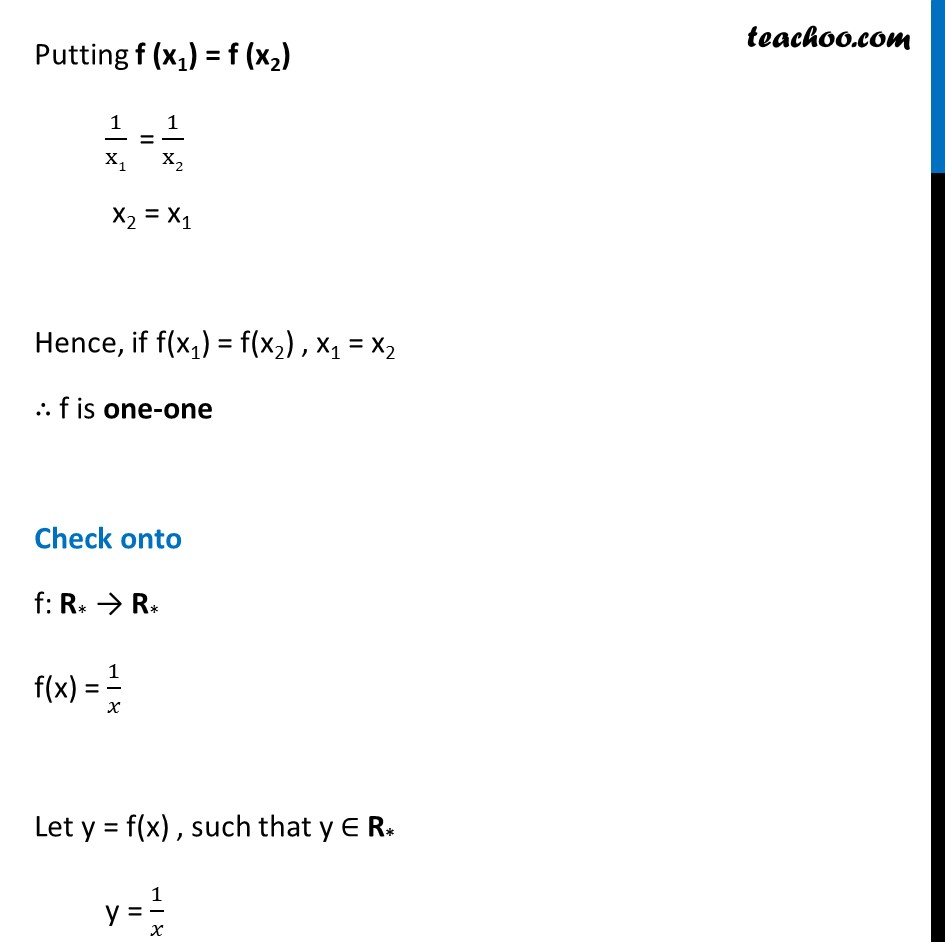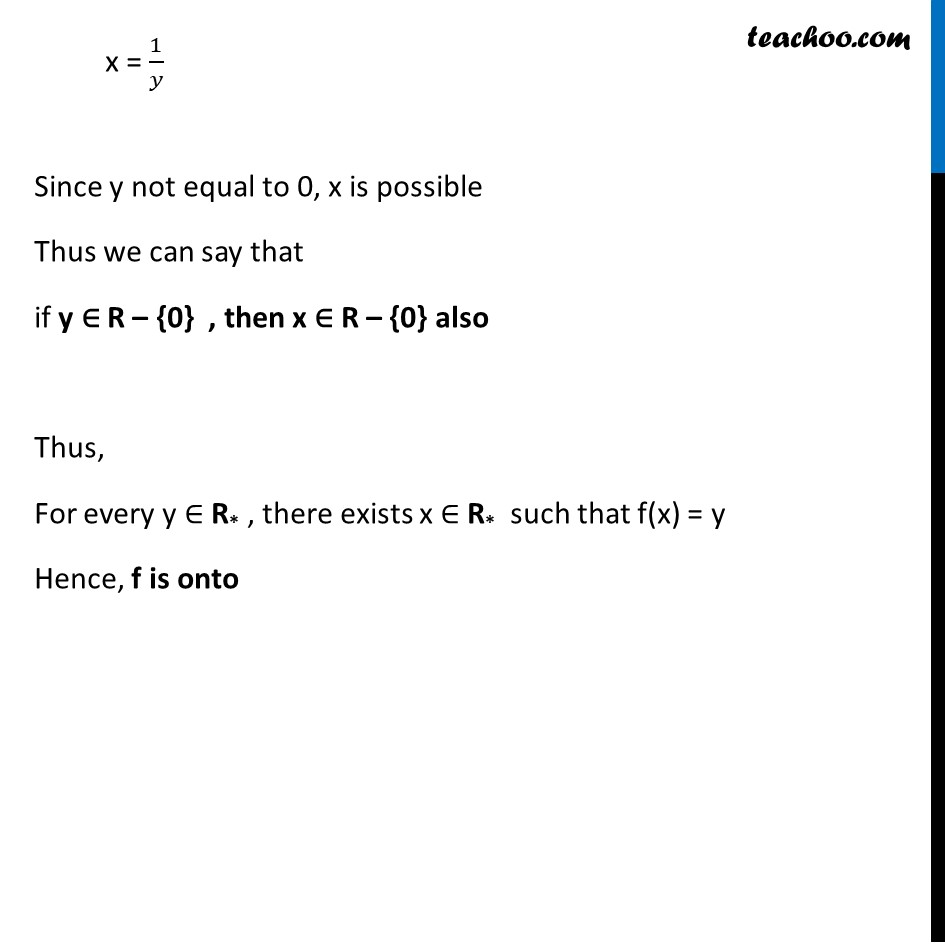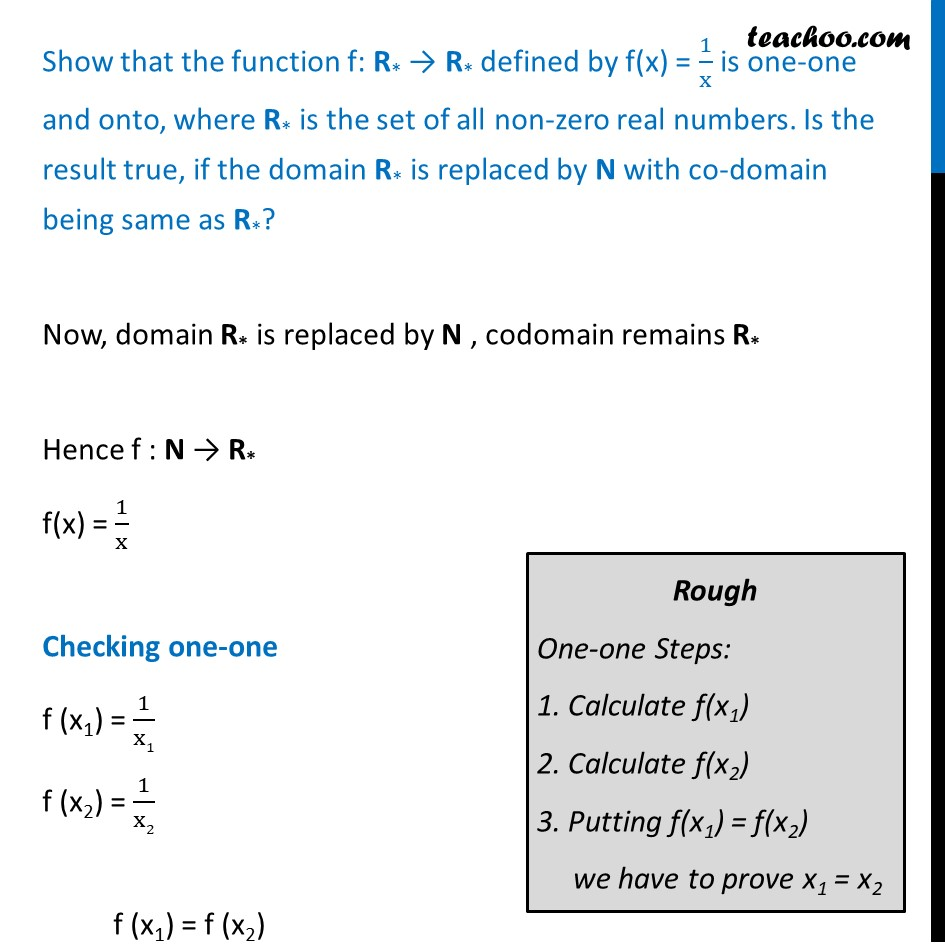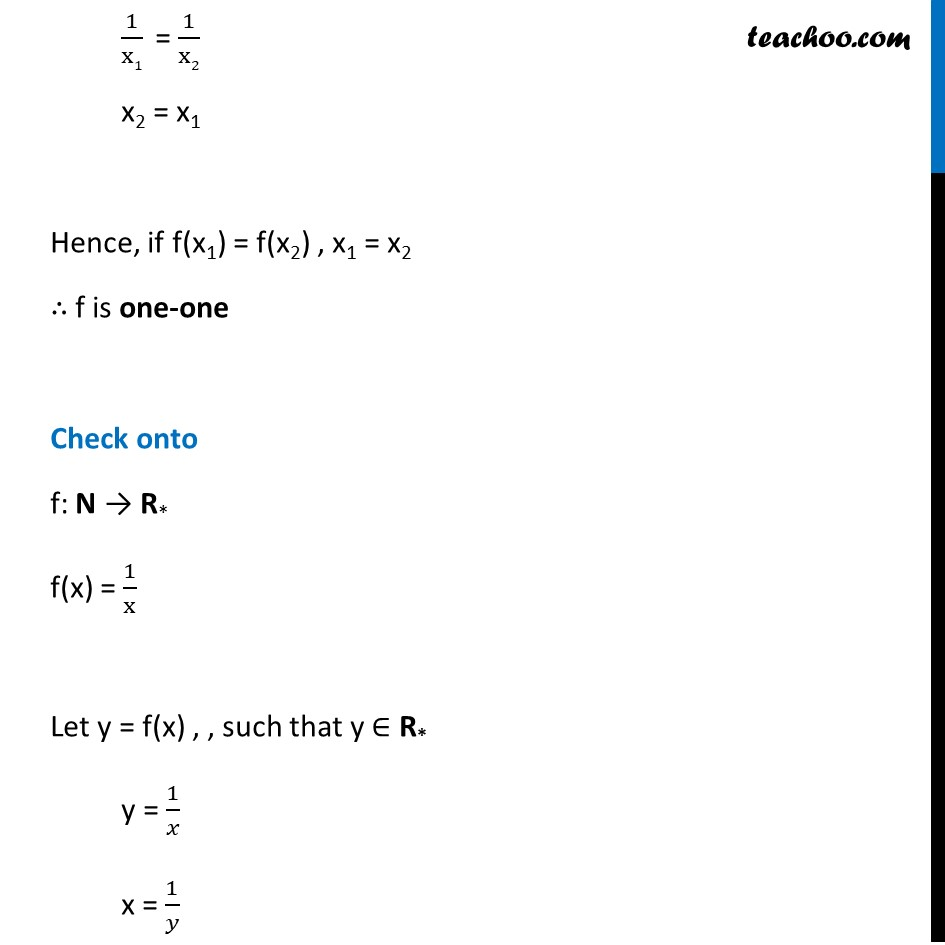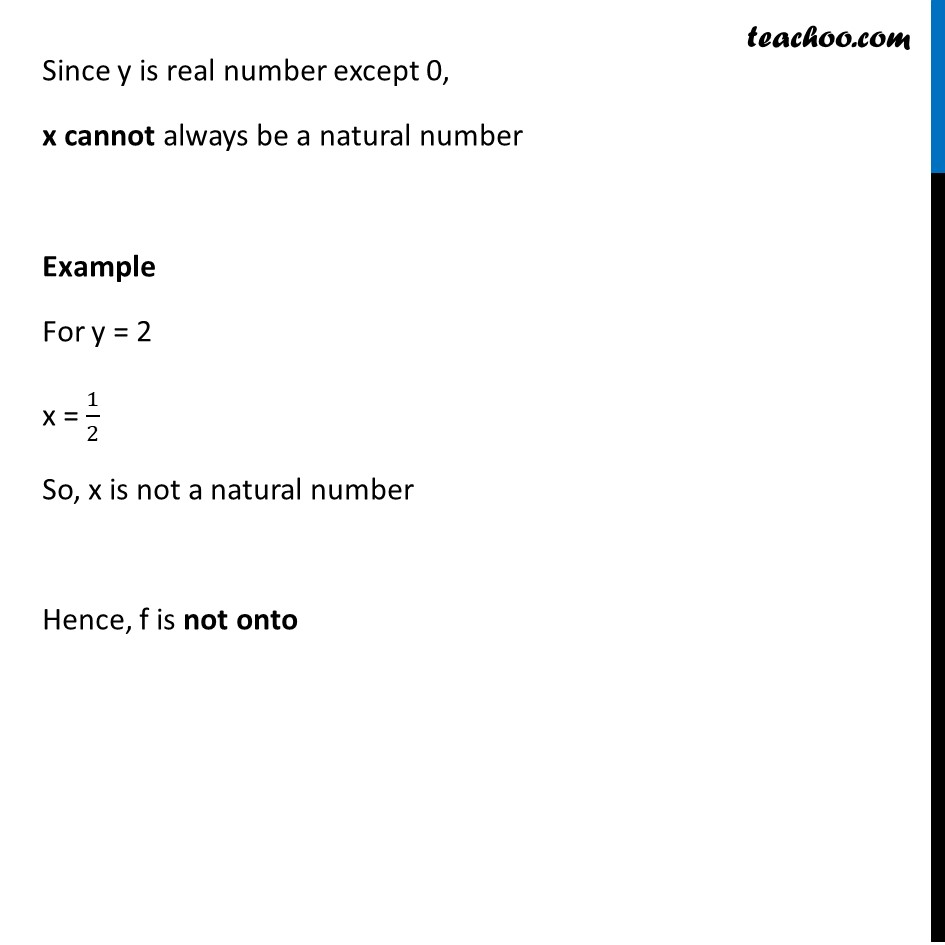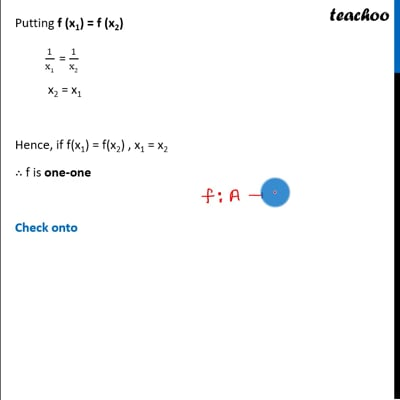This video is only available for Teachoo black usersThis video is only available for Teachoo black users

Learn in your speed, with individual attention - Teachoo Maths 1-on-1 Class

### Transcript

Ex 1.2, 1 Show that the function f: R* → R* defined by f(x) = 1/x is one-one and onto, where R* is the set of all non-zero real numbers. Is the result true, if the domain R* is replaced by N with co-domain being same as R*? Solving for f: R* → R* f(x) = 1/x Checking one-one f (x1) = 1/𝑥1 f (x2) = 1/𝑥2 Putting f (x1) = f (x2) 1/x1 = 1/x2 x2 = x1 Hence, if f(x1) = f(x2) , x1 = x2 ∴ f is one-one Check onto f: R* → R* f(x) = 1/𝑥 Let y = f(x) , such that y ∈ R* y = 1/𝑥 x = 1/𝑦 Since y not equal to 0, x is possible Thus we can say that if y ∈ R – {0} , then x ∈ R – {0} also Thus, For every y ∈ R* , there exists x ∈ R* such that f(x) = y Hence, f is onto Show that the function f: R* → R* defined by f(x) = 1/x is one-one and onto, where R* is the set of all non-zero real numbers. Is the result true, if the domain R* is replaced by N with co-domain being same as R*? Now, domain R* is replaced by N , codomain remains R* Hence f : N → R* f(x) = 1/x Checking one-one f (x1) = 1/x1 f (x2) = 1/x2 f (x1) = f (x2) 1/x1 = 1/x2 x2 = x1 Hence, if f(x1) = f(x2) , x1 = x2 ∴ f is one-one Check onto f: N → R* f(x) = 1/x Let y = f(x) , , such that y ∈ R* y = 1/𝑥 x = 1/𝑦Since y is real number except 0, x cannot always be a natural number Example For y = 2 x = 1/2 So, x is not a natural number Hence, f is not onto